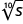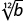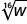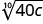# 9.8 Rational exponents  (Page 4/7)

 Page 4 / 7

Simplify: ${\left(16{m}^{\frac{1}{3}}\right)}^{\frac{3}{2}}$ ${\left(81{n}^{\frac{2}{5}}\right)}^{\frac{3}{2}}$ .

$64{m}^{\frac{1}{2}}$ $729{n}^{\frac{3}{5}}$

Simplify: ${\left({m}^{3}{n}^{9}\right)}^{\frac{1}{3}}$ ${\left({p}^{4}{q}^{8}\right)}^{\frac{1}{4}}$ .

## Solution

1. $\begin{array}{cccc}& & & {\left({m}^{3}{n}^{9}\right)}^{\frac{1}{3}}\hfill \\ \\ \\ \begin{array}{c}\text{First we use the Product to a Power}\hfill \\ \text{Property.}\hfill \end{array}\hfill & & & {\left({m}^{3}\right)}^{\frac{1}{3}}{\left({n}^{9}\right)}^{\frac{1}{3}}\hfill \\ \\ \\ \begin{array}{c}\text{To raise a power to a power, we multiply}\hfill \\ \text{the exponents.}\hfill \end{array}\hfill & & & m{n}^{3}\hfill \end{array}$

2. $\begin{array}{cccc}& & & {\left({p}^{4}{q}^{8}\right)}^{\frac{1}{4}}\hfill \\ \\ \\ \begin{array}{c}\text{First we use the Product to a Power}\hfill \\ \text{Property.}\hfill \end{array}\hfill & & & {\left({p}^{4}\right)}^{\frac{1}{4}}{\left({q}^{8}\right)}^{\frac{1}{4}}\hfill \\ \\ \\ \begin{array}{c}\text{To raise a power to a power, we multiply}\hfill \\ \text{the exponents.}\hfill \end{array}\hfill & & & p{q}^{2}\hfill \end{array}$

We will use both the Product and Quotient Properties in the next example.

Simplify: $\frac{{x}^{\frac{3}{4}}·{x}^{-\frac{1}{4}}}{{x}^{-\frac{6}{4}}}$ $\frac{{y}^{\frac{4}{3}}·y}{{y}^{-\frac{2}{3}}}$ .

## Solution

1. $\begin{array}{cccc}& & & \frac{{x}^{\frac{3}{4}}·{x}^{-\frac{1}{4}}}{{x}^{-\frac{6}{4}}}\hfill \\ \\ \\ \begin{array}{c}\text{Use the Product Property in the numerator,}\hfill \\ \text{add the exponents.}\hfill \end{array}\hfill & & & \frac{{x}^{\frac{2}{4}}}{{x}^{-\frac{6}{4}}}\hfill \\ \\ \\ \begin{array}{c}\text{Use the Quotient Property, subtract the}\hfill \\ \text{exponents.}\hfill \end{array}\hfill & & & {x}^{\frac{8}{4}}\hfill \\ \text{Simplify.}\hfill & & & {x}^{2}\hfill \end{array}$

2. $\begin{array}{cccc}& & & \frac{{y}^{\frac{4}{3}}·y}{{y}^{-\frac{2}{3}}}\hfill \\ \\ \\ \begin{array}{c}\text{Use the Product Property in the numerator,}\hfill \\ \text{add the exponents.}\hfill \end{array}\hfill & & & \frac{{y}^{\frac{7}{3}}}{{y}^{-\frac{2}{3}}}\hfill \\ \\ \\ \begin{array}{c}\text{Use the Quotient Property, subtract the}\hfill \\ \text{exponents.}\hfill \end{array}\hfill & & & {y}^{\frac{9}{3}}\hfill \\ \\ \\ \text{Simplify.}\hfill & & & {y}^{3}\hfill \end{array}$

Simplify: $\frac{{m}^{\frac{2}{3}}·{m}^{-\frac{1}{3}}}{{m}^{-\frac{5}{3}}}$ $\frac{{n}^{\frac{1}{6}}·n}{{n}^{-\frac{11}{6}}}$ .

${m}^{2}$ ${n}^{3}$

Simplify: $\frac{{u}^{\frac{4}{5}}·{u}^{-\frac{2}{5}}}{{u}^{-\frac{13}{5}}}$ $\frac{{v}^{\frac{1}{2}}·v}{{v}^{-\frac{7}{2}}}$ .

${u}^{3}$ ${v}^{5}$

## Key concepts

• Summary of Exponent Properties
• If $a,b$ are real numbers and $m,n$ are rational numbers, then
• Product Property ${a}^{m}·{a}^{n}={a}^{m+n}$
• Power Property ${\left({a}^{m}\right)}^{n}={a}^{m·n}$
• Product to a Power ${\left(ab\right)}^{m}={a}^{m}{b}^{m}$
• Quotient Property :
$\frac{{a}^{m}}{{a}^{n}}={a}^{m-n},\phantom{\rule{0.2em}{0ex}}\text{}\phantom{\rule{0.2em}{0ex}}a\ne 0,\phantom{\rule{0.2em}{0ex}}\text{}\phantom{\rule{0.2em}{0ex}}m>n$
$\frac{{a}^{m}}{{a}^{n}}=\frac{1}{{a}^{n-m}},\phantom{\rule{0.2em}{0ex}}\text{}\phantom{\rule{0.2em}{0ex}}a\ne 0,\phantom{\rule{0.2em}{0ex}}\text{}\phantom{\rule{0.2em}{0ex}}n>m$
• Zero Exponent Definition ${a}^{0}=1$ , $a\ne 0$
• Quotient to a Power Property ${\left(\frac{a}{b}\right)}^{m}=\frac{{a}^{m}}{{b}^{m}},\phantom{\rule{0.5em}{0ex}}b\ne 0$

## Practice makes perfect

Simplify Expressions with ${a}^{\frac{1}{n}}$

In the following exercises, write as a radical expression.

${x}^{\frac{1}{2}}$
${y}^{\frac{1}{3}}$
${z}^{\frac{1}{4}}$

${r}^{\frac{1}{2}}$
${s}^{\frac{1}{3}}$
${t}^{\frac{1}{4}}$

$\sqrt{r}$ $\sqrt{s}$ $\sqrt{t}$

${u}^{\frac{1}{5}}$
${v}^{\frac{1}{9}}$
${w}^{\frac{1}{20}}$

${g}^{\frac{1}{7}}$
${h}^{\frac{1}{5}}$
${j}^{\frac{1}{25}}$

$\sqrt{g}$ $\sqrt{h}$ $\sqrt{j}$

In the following exercises, write with a rational exponent.

$-\sqrt{x}$
$\sqrt{y}$
$\sqrt{f}$

$\sqrt{r}$$\sqrt{t}$

${r}^{\frac{1}{8}}$ ${s}^{\frac{1}{10}}$ ${t}^{\frac{1}{4}}$

$\sqrt{a}$$\sqrt{c}$

$\sqrt{u}$
$\sqrt{v}$${u}^{\frac{1}{5}}$ ${v}^{\frac{1}{2}}$ ${w}^{\frac{1}{16}}$

$\sqrt{7c}$
$\sqrt{12d}$
$3\sqrt{5f}$

$\sqrt{5x}$
$\sqrt{9y}$
$7\sqrt{3z}$

${\left(5x\right)}^{\frac{1}{4}}$ ${\left(9y\right)}^{\frac{1}{8}}$ $7{\left(3z\right)}^{\frac{1}{5}}$

$\sqrt{21p}$
$\sqrt{8q}$
$4\sqrt{36r}$

$\sqrt{25a}$
$\sqrt{3b}$${\left(25a\right)}^{\frac{1}{3}}$ ${\left(3b\right)}^{\frac{1}{2}}$ ${\left(40c\right)}^{\frac{1}{10}}$

In the following exercises, simplify.

${81}^{\frac{1}{2}}$
${125}^{\frac{1}{3}}$
${64}^{\frac{1}{2}}$

${625}^{\frac{1}{4}}$
${243}^{\frac{1}{5}}$
${32}^{\frac{1}{5}}$

5 3 2

${16}^{\frac{1}{4}}$
${16}^{\frac{1}{2}}$
${3125}^{\frac{1}{5}}$

${216}^{\frac{1}{3}}$
${32}^{\frac{1}{5}}$
${81}^{\frac{1}{4}}$

6 2 3

${\left(-216\right)}^{\frac{1}{3}}$
$\text{−}{216}^{\frac{1}{3}}$
${\left(216\right)}^{-\frac{1}{3}}$

${\left(-243\right)}^{\frac{1}{5}}$
$\text{−}{243}^{\frac{1}{5}}$
${\left(243\right)}^{-\frac{1}{5}}$

$-3$ $-3$ $\frac{1}{3}$

${\left(-1\right)}^{\frac{1}{3}}$
${-1}^{\frac{1}{3}}$
${\left(1\right)}^{-\frac{1}{3}}$

${\left(-1000\right)}^{\frac{1}{3}}$
$\text{−}{1000}^{\frac{1}{3}}$
${\left(1000\right)}^{-\frac{1}{3}}$

$-10$ $-10$ $\frac{1}{10}$

${\left(-81\right)}^{\frac{1}{4}}$
$\text{−}{81}^{\frac{1}{4}}$
${\left(81\right)}^{-\frac{1}{4}}$

${\left(-49\right)}^{\frac{1}{2}}$
$\text{−}{49}^{\frac{1}{2}}$
${\left(49\right)}^{-\frac{1}{2}}$

not a real number $-7$ $\frac{1}{7}$

${\left(-36\right)}^{\frac{1}{2}}$
$-{36}^{\frac{1}{2}}$
${\left(36\right)}^{-\frac{1}{2}}$

${\left(-1\right)}^{\frac{1}{4}}$
${\left(1\right)}^{-\frac{1}{4}}$
$\text{−}{1}^{\frac{1}{4}}$

not a real number $1$ $-1$

${\left(-100\right)}^{\frac{1}{2}}$
$\text{−}{100}^{\frac{1}{2}}$
${\left(100\right)}^{-\frac{1}{2}}$

${\left(-32\right)}^{\frac{1}{5}}$
${\left(243\right)}^{-\frac{1}{5}}$
$\text{−}{125}^{\frac{1}{3}}$

$-2$ $\frac{1}{3}$
$-5$

Simplify Expressions with ${a}^{\frac{m}{n}}$

In the following exercises, write with a rational exponent.

$\sqrt{{m}^{5}}$
$\sqrt{{n}^{2}}$
$\sqrt{{p}^{3}}$

$\sqrt{{r}^{7}}$
$\sqrt{{s}^{3}}$
$\sqrt{{t}^{7}}$

${r}^{\frac{7}{4}}$ ${s}^{\frac{3}{5}}$ ${t}^{\frac{7}{3}}$

$\sqrt{{u}^{2}}$
$\sqrt{{v}^{8}}$
$\sqrt{{w}^{4}}$

$\sqrt{a}$
$\sqrt{{b}^{5}}$
$\sqrt{{c}^{5}}$

${a}^{\frac{1}{3}}$ ${b}^{\frac{1}{5}}$ ${c}^{\frac{5}{3}}$

In the following exercises, simplify.

${16}^{\frac{3}{2}}$
${8}^{\frac{2}{3}}$
${10,000}^{\frac{3}{4}}$

${1000}^{\frac{2}{3}}$
${25}^{\frac{3}{2}}$
${32}^{\frac{3}{5}}$

100 125 8

${27}^{\frac{5}{3}}$
${16}^{\frac{5}{4}}$
${32}^{\frac{2}{5}}$

${16}^{\frac{3}{2}}$
${125}^{\frac{5}{3}}$
${64}^{\frac{4}{3}}$

64 3125 256

${32}^{\frac{2}{5}}$
${27}^{-\frac{2}{3}}$
${25}^{-\frac{3}{2}}$

${64}^{\frac{5}{2}}$
${81}^{-\frac{3}{2}}$
${27}^{-\frac{4}{3}}$

32,768 $\frac{1}{729}$ $\frac{1}{81}$

${25}^{\frac{3}{2}}$
${9}^{-\frac{3}{2}}$
${\left(-64\right)}^{\frac{2}{3}}$

${100}^{\frac{3}{2}}$
${49}^{-\frac{5}{2}}$
${\left(-100\right)}^{\frac{3}{2}}$

1000 $\frac{1}{16,807}$ not a real number

$\text{−}{9}^{\frac{3}{2}}$
$\text{−}{9}^{-\frac{3}{2}}$
${\left(-9\right)}^{\frac{3}{2}}$

$\text{−}{64}^{\frac{3}{2}}$
$\text{−}{64}^{-\frac{3}{2}}$
${\left(-64\right)}^{\frac{3}{2}}$

$-512$
$-\frac{1}{512}$ not a real number

$\text{−}{100}^{\frac{3}{2}}$
$\text{−}{100}^{-\frac{3}{2}}$
${\left(-100\right)}^{\frac{3}{2}}$

$\text{−}{49}^{\frac{3}{2}}$
$\text{−}{49}^{-\frac{3}{2}}$
${\left(-49\right)}^{\frac{3}{2}}$

$-343$ $-\frac{1}{343}$ not a real number

Use the Laws of Exponents to Simplify Expressions with Rational Exponents

In the following exercises, simplify.

${4}^{\frac{5}{8}}·{4}^{\frac{11}{8}}$
${m}^{\frac{7}{12}}·{m}^{\frac{17}{12}}$
${p}^{\frac{3}{7}}·{p}^{\frac{18}{7}}$

${6}^{\frac{5}{2}}·{6}^{\frac{1}{2}}$
${n}^{\frac{2}{10}}·{n}^{\frac{8}{10}}$
${q}^{\frac{2}{5}}·{q}^{\frac{13}{5}}$

216 $n$ ${q}^{3}$

${5}^{\frac{1}{2}}·{5}^{\frac{7}{2}}$
${c}^{\frac{3}{4}}·{c}^{\frac{9}{4}}$
${d}^{\frac{3}{5}}·{d}^{\frac{2}{5}}$

${10}^{\frac{1}{3}}·{10}^{\frac{5}{3}}$
${x}^{\frac{5}{6}}·{x}^{\frac{7}{6}}$
${y}^{\frac{11}{8}}·{y}^{\frac{21}{8}}$

100 ${x}^{2}$ ${y}^{4}$

${\left({m}^{6}\right)}^{\frac{5}{2}}$
${\left({n}^{9}\right)}^{\frac{4}{3}}$
${\left({p}^{12}\right)}^{\frac{3}{4}}$

${\left({a}^{12}\right)}^{\frac{1}{6}}$
${\left({b}^{15}\right)}^{\frac{3}{5}}$
${\left({c}^{11}\right)}^{\frac{1}{11}}$

${a}^{2}$ ${b}^{9}$
$c$

${\left({x}^{12}\right)}^{\frac{2}{3}}$
${\left({y}^{20}\right)}^{\frac{2}{5}}$
${\left({z}^{16}\right)}^{\frac{1}{16}}$

${\left({h}^{6}\right)}^{\frac{4}{3}}$
${\left({k}^{12}\right)}^{\frac{3}{4}}$
${\left({j}^{10}\right)}^{\frac{7}{5}}$

${h}^{8}$ ${k}^{9}$ ${j}^{14}$

$\frac{{x}^{\frac{7}{2}}}{{x}^{\frac{5}{2}}}$
$\frac{{y}^{\frac{5}{2}}}{{y}^{\frac{1}{2}}}$
$\frac{{r}^{\frac{4}{5}}}{{r}^{\frac{9}{5}}}$

$\frac{{s}^{\frac{11}{5}}}{{s}^{\frac{6}{5}}}$
$\frac{{z}^{\frac{7}{3}}}{{z}^{\frac{1}{3}}}$
$\frac{{w}^{\frac{2}{7}}}{{w}^{\frac{9}{7}}}$

$s$ ${z}^{2}$ $\frac{1}{w}$

$\frac{{t}^{\frac{12}{5}}}{{t}^{\frac{7}{5}}}$
$\frac{{x}^{\frac{3}{2}}}{{x}^{\frac{1}{2}}}$
$\frac{{m}^{\frac{13}{8}}}{{m}^{\frac{5}{8}}}$

$\frac{{u}^{\frac{13}{9}}}{{u}^{\frac{4}{9}}}$
$\frac{{r}^{\frac{15}{7}}}{{r}^{\frac{8}{7}}}$
$\frac{{n}^{\frac{3}{5}}}{{n}^{\frac{8}{5}}}$

$u$ $r$ $\frac{1}{n}$

${\left(9{p}^{\frac{2}{3}}\right)}^{\frac{5}{2}}$
${\left(27{q}^{\frac{3}{2}}\right)}^{\frac{4}{3}}$

${\left(81{r}^{\frac{4}{5}}\right)}^{\frac{1}{4}}$
${\left(64{s}^{\frac{3}{7}}\right)}^{\frac{1}{6}}$

$3{r}^{\frac{1}{5}}$ $2{s}^{\frac{1}{14}}$

${\left(16{u}^{\frac{1}{3}}\right)}^{\frac{3}{4}}$
${\left(100{v}^{\frac{2}{5}}\right)}^{\frac{3}{2}}$

${\left(27{m}^{\frac{3}{4}}\right)}^{\frac{2}{3}}$
${\left(625{n}^{\frac{8}{3}}\right)}^{\frac{3}{4}}$

$9{m}^{\frac{1}{2}}$ $125{n}^{2}$

Can you explain further
How do you find divisible numbers without a calculator?
TAKE OFF THE LAST DIGIT AND MULTIPLY IT 9. SUBTRACT IT THE DIGITS YOU HAVE LEFT. IF THE ANSWER DIVIDES BY 13(OR IS ZERO), THEN YOUR ORIGINAL NUMBER WILL ALSO DIVIDE BY 13!IS DIVISIBLE BY 13
BAINAMA
When she graduates college, Linda will owe $43,000 in student loans. The interest rate on the federal loans is 4.5% and the rate on the private bank loans is 2%. The total interest she owes for one year was$1,585. What is the amount of each loan?
Sean took the bus from Seattle to Boise, a distance of 506 miles. If the trip took 7 2/3 hours, what was the speed of the bus?
66miles/hour
snigdha
How did you work it out?
Esther
s=mi/hr 2/3~0.67 s=506mi/7.67hr = ~66 mi/hr
Orlando
hello, I have algebra phobia. Subtracting negative numbers always seem to get me confused.
what do you need help in?
Felix
Heather
look at the numbers if they have different signs, it's like subtracting....but you keep the sign of the largest number...
Felix
for example.... -19 + 7.... different signs...subtract.... 12 keep the sign of the "largest" number 19 is bigger than 7.... 19 has the negative sign... Therefore, -12 is your answer...
Felix
—12
Thanks Felix.l also get confused with signs.
Esther
Thank you for this
Shatey
ty
Graham
think about it like you lost $19 (-19), then found$7(+7). Totally you lost just $12 (-12) Annushka I used to struggle a lot with negative numbers and math in general what I typically do is look at it in terms of money I have -$5 in my account I then take out 5 more dollars how much do I have in my account well-\$10 ... I also for a long time would draw it out on a number line to visualize it
Meg
practicing with smaller numbers to understand then working with larger numbers helps too and the song/rhyme same sign add and keep opposite signs subtract keep the sign of the bigger # then you'll be exact
Meg
Bruce drives his car for his job. The equation R=0.575m+42 models the relation between the amount in dollars, R, that he is reimbursed and the number of miles, m, he drives in one day. Find the amount Bruce is reimbursed on a day when he drives 220 miles
168.50=R
Heather
john is 5years older than wanjiru.the sum of their years is27years.what is the age of each
46
mustee
j 17 w 11
Joseph
john is 16. wanjiru is 11.
Felix
27-5=22 22÷2=11 11+5=16
Joyce
I don't see where the answers are.
Ed
Cindy and Richard leave their dorm in Charleston at the same time. Cindy rides her bicycle north at a speed of 18 miles per hour. Richard rides his bicycle south at a speed of 14 miles per hour. How long will it take them to be 96 miles apart?
3
Christopher
18t+14t=96 32t=96 32/96 3
Christopher
show that a^n-b^2n is divisible by a-b
What does 3 times your weight right now
Use algebra to combine 39×5 and the half sum of travel of 59+30
Cherokee
What is the segment of 13? Explain
Cherokee
my weight is 49. So 3 times is 147
Cherokee
kg to lbs you goin to convert 2.2 or one if the same unit your going to time your body weight by 3. example if my body weight is 210lb. what would be my weight if I was 3 times as much in kg. that's you do 210 x3 = 630lb. then 630 x 2.2= .... hope this helps
tyler
How to convert grams to pounds?
paul
What is the lcm of 340
Yes
Cherokee
How many numbers each equal to y must be taken to make 15xy
15x
Martin
15x
Asamoah
15x
Hugo
1y
Tom
1y x 15y
Tom
find the equation whose roots are 1 and 2
(x - 2)(x -1)=0 so equation is x^2-x+2=0
Ranu
I believe it's x^2-3x+2
NerdNamedGerg
because the X's multiply by the -2 and the -1 and than combine like terms
NerdNamedGerg
find the equation whose roots are -1 and 4
Ans = ×^2-3×+2
Gee
find the equation whose roots are -2 and -1
(×+1)(×-4) = x^2-3×-4
Gee
yeah
Asamoah
there's a chatting option in the app wow
Nana
That's cool cool
Nana
Nice to meet you all
Nana
you too.
Joan
😃
Nana
Hey you all there are several Free Apps that can really help you to better solve type Equations.
Debra
Debra, which apps specifically. ..?
Nana
am having a course in elementary algebra ,any recommendations ?
samuel
Samuel Addai, me too at ucc elementary algebra as part of my core subjects in science
Nana
me too as part of my core subjects in R M E
Ken
at ABETIFI COLLEGE OF EDUCATION
Ken
ok great. Good to know.
Joan
5x + 1/3= 2x + 1/2
sanam
Plz solve this
sanam
5x - 3x = 1/2 - 1/3 2x = 1/6 x = 1/12
Ranu
Thks ranu
sanam
Erica
the previous equation should be 3x = 1/6 x=1/18
Sriram
for the new one 10x + 2x = 38 - 14
Sriram
12x = 24 x=2
Sriram
10x + 14 = -2x +38 10x + 2x = 38 - 14 12x = 24 divide both sides by the coefficient of x, which is 12 therefore × = 2
vidaByByBy